# ML Aggarwal Class 7 Solutions for ICSE Maths Chapter 1 Integers Objective Type Questions

## ML Aggarwal Class 7 Solutions for ICSE Maths Chapter 1 Integers Objective Type Questions

Mental Maths

Question 1.
Fill in the blanks:
(i) ………… is the greatest negative integer.
(ii) ((-10) + 3) + (-12) = (-10) + (3 + …..)
(iii) The product of two negative integers and the product of two positive integers is a ………. integer.
(iv) The division of any integer by zero is ……..
(v) The integer whose product with (-1) is 22 is ………
(vi) (-15) × …….. = 120
(vii) ……… ÷ (-6) = -12
(viii) (-10) × ((-15) + 33) = ……. × (-15) + (-10) × 33
(ix) ……… ÷ (-25) = 0
(x) ((-8) × (-13)) × 27 = (-8) × ((……) × 27)
(xi) 13 × (-6) = -(……. × ………) = …….
(xii) (-a) + b = b + additive inverse of ……….
(xiii) When we divide a negative integer by a positive integer, we divide them as whole numbers and put a ……… sign before the quotient.
(xiv) When -25 is divided by ……… the quotient is 5.
(xv) There are …….. pairs of integers satisfying a ÷ b = -1.
(xvi) The value of the expression ((-60) ÷ 12) (-5) is ……….
Solution: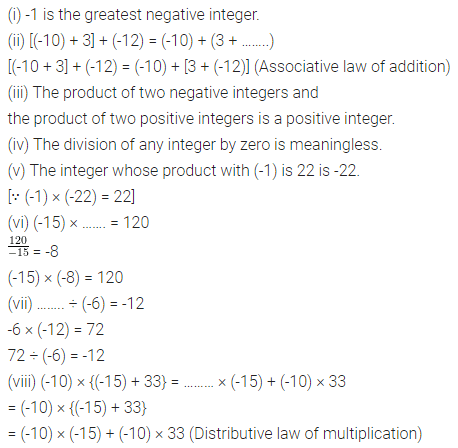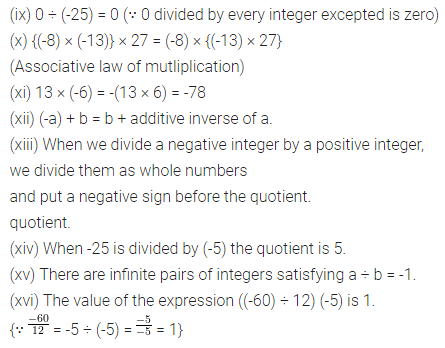Question 2.
State whether the following statements are True (T) or False (F):
(i) For every integer a, |a| is either positive or zero.
(ii) The difference of two negative integers cannot be a positive integer.
(iii) We can write a pair of integers whose sum is not an integer.
(iv) Going 300 metres towards east first and then 100 m back is the same as going 100 m towards west first and then going 300 back.
(v) If we multiply an integer by (-1), then the result is the additive inverse of the integer.
(vi) If we divide an integer by (-1), then the result is the additive inverse of the integer.
(vii) 1 is the additive identity of integers.
(viii) (-17) × 6 is a whole number.
(ix) (-5) × (-8) × o is a positive integer.
(x) (-237) × 0 is same as 0 × (-89).
(xi) The product of 5 negative integers is a negative integer.
(xii) Closure property holds for subtraction of integers.
(xiii) Commutative property does not hold for subtraction of integers.
(xiv) Associative property holds for subtraction of integers.
(xv) Closure property holds for division of integers.
(xvi) Commutative property does not hold for division of integers.
(xvii) (-1) is not a mutliplicative identity of integers.
(xviii) Multiplication fact (-8) × (-12) = 96 is same as division fact 96 ÷ (-12) = -8.
(xix) [(-32 ÷ 8] ÷ 2 = (-32) ÷ (8 ÷ 2)
(xx) For every integer a, a ÷ a = 1.
(xxi) The successor of 0 × (-10) is 1 × (-10).
Solution: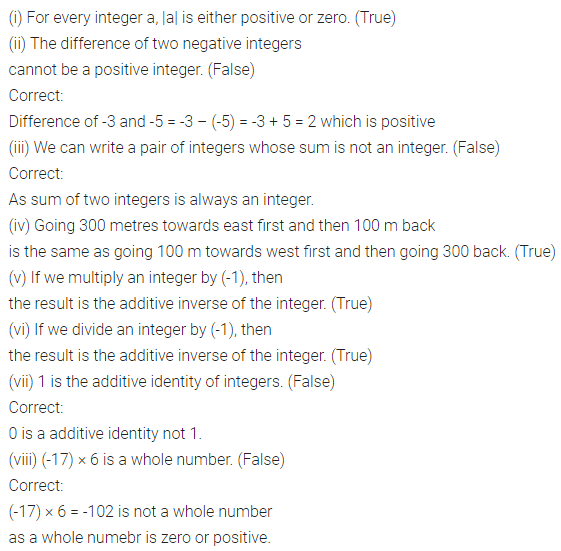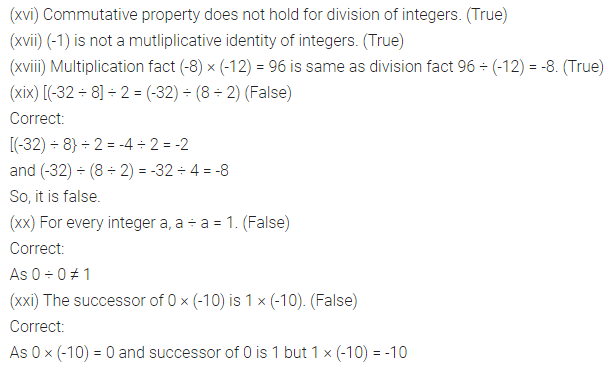Question 3.
State whether the following statements are true or false. Justify your answer.
(i) The sum of a positive integer and a negative integer is always a positive integer.
(ii) The sum of two integers is always greater than their difference.
(iii) For any two integers a and b, the inequality -a < b is always true.
(iv) The product of two integers is always greater than the sum of the integers.
Solution:Multiple Choice Questions

Choose the correct answer from the given four options (4 to 19):
Question 4.
If the integers 10, -7, 5, 3, -4 and 0 are marked on the number line, then the integer which lies on the extreme left is
(a) 10
(b) 0
(c) -7
(d) -4
Solution: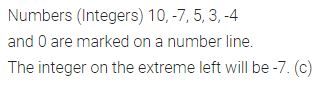Question 5.
On the number line, the value of (-3) × 3 lies on the right hand side of
(a) -10
(b) -6
(c) 0
(d) 9
Solution: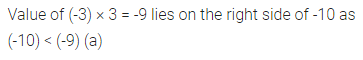Question 6.
The vqlue of 5 ÷ (-1) does not lie between
(a) 0 and-10
(b) Oand 10
(c) -3 and -10
(d) -7 and 7
Solution: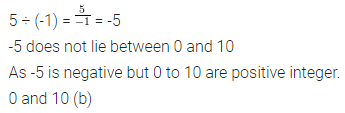Question 7.
The next number in the pattern -62, -37, -12, ……. is
(a) 25
(b) 0
(c) 13
(d) -13
Solution:Question 8.
Multiplication of integers satisfies the property of
(a) closure
(b) commutativity
(c) associativity
(d) all of these
Solution:Question 9.
Closure property does not hold in integers for
(a) multiplication
(b) division
(d) subtraction
Solution:Question 10.
The number of integers between -20 and -10 are
(a) 8
(b) 9
(c) 10
(d) 11
Solution:Question 11.
If the sum of two integers is -10 and one of them is 2, then the other is
(a) 8
(b) -8
(b) 12
(d) -12
Solution:Question 12.
The integer that must be subtracted from -5 to obtain -12 is
(a) 7
(b) -7
(c) 17
(d) -17
Solution: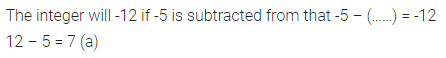Question 13.
Which of the following is not the additive inverse of a?
(a) -(-a)
(b) -a
(c) a ÷ (-1)
(d) a × (-1)
Solution:Question 14.
0 ÷ (-10) is equal to
(a) 0
(b) -1
(c) -10
(d) none of these
Solution:Question 15.
(-33) × 102 + (-33) × (-2) is equal to
(a) 3300
(b) -3300
(c) 3432
(d) -3432
Solution: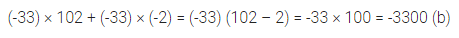Question 16.
(-25) (6 + 4) is not the same as
(a) -250
(b) (-25) × 10
(c) (-25) × 6 × 4
(d) (-25) × 6 + (-25) × 4
Solution: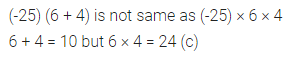Question 17.
101 × (-1) + 0 ÷ (-1) is equal to
(a) -101
(b) 101
(c) -102
(d) 102
Solution:Question 18.
If a and b are two integers, then which of the following may not be an integer?
(a) a + b
(b) a – b
(c) a × b
(d) a ÷ b
Solution: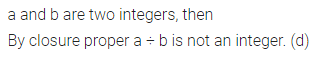Question 19.
For a non-zero integer a which is the following is not defined?
(a) a ÷ 0
(b) 0 ÷ a
(c) a = 1
(d) 1 ÷ a
Solution: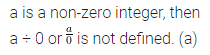Value Based Questions

Question 1.
In a competitive exam, 3 marks are given for every correct answer and 1 mark is deducted for every incorrect answer. Raju copied some answers from Reema and answered all the questions, he scored 20 marks though he got 10 correct answers. How many incorrect answers had he attempted? What values are promoted in the question?
Solution:Question 2.
In a quiz, ₹ 300 are awarded for every correct answer and a penalty of ₹ 75 is put for every incorrect answer. Madhuri answered 15 questions out of which only 6 answers were correct. How much money is earned by Madhuri in the quiz?
If she distributes the money earned by her to poor children in the neighbourhood, what values are being promoted?
Solution:Higher Order Thinking Skills (HOTS)

Question 1.
Write a pair of integers whose product is -12 and there lies seven integers between them.
Solution:Question 2.
A water tank has steps inside it. A monkey is sitting on the first step. The water level is at the ninth step.
(i) He jumps 3 steps down and then jumps back 2 steps up. In how many jumps will he reach the water level?
(ii) After drinking water, he wants to go back. For this, he jumps 5 steps up and then jumps back 3 steps down in every move. In how many jumps will he reach back the top of the tank?
Solution: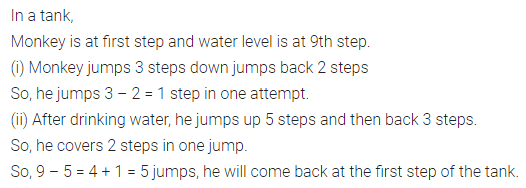Question 3.
A shopkeeper earns a profit of ₹ 2 by selling a pen and incurs a loss of 50 paise per pencil and loss of 15 paise per eraser while selling pencils and erasers of old stock. On a particular day, he earns a profit of ₹ 10. If he sold 10 pens and the number of pencils and erasers he sold are in the ratio 7 : 10, then find the number of pencils and eraser she sold on that day.
Solution: